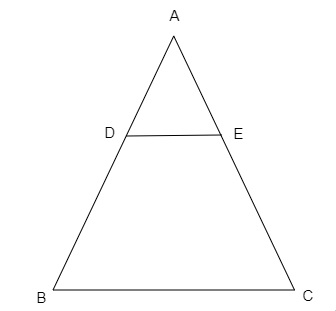# $D$ and $E$ are the points on the sides $AB$ and $AC$ respectively of a $\triangle ABC$ such that: $AD = 8\ cm, DB = 12\ cm, AE = 6\ cm$ and $CE = 9\ cm$. Prove that $BC = \frac{5}{2}DE$.

Given:

$D$ and $E$ are the points on the sides $AB$ and $AC$ respectively of a $\triangle ABC$ such that: $AD = 8\ cm, DB = 12\ cm, AE = 6\ cm$ and $CE = 9\ cm$.

To do:

We have to prove that $BC = \frac{5}{2}DE$.

Solution:In $\triangle ADE$ and $\triangle ABC$,

$\frac{AD}{DB}=\frac{8}{12}=\frac{2}{3}$

$\frac{AE}{EC}=\frac{6}{9}=\frac{2}{3}$

$\frac{AD}{DB}=\frac{AE}{EC}$

By converse of basic proportionality theorem,

$DE \parallel BC$

In $\triangle ADE$ and $\triangle ABC$,

$\angle A=\angle A$

$\angle ADE=\angle ABC$

Therefore,

$\triangle ADE \sim\ \triangle ABC$   (By AA similarity)

$\frac{AD}{AB}=\frac{DE}{BC}$   (CPCT)

$\frac{8}{8+12}=\frac{DE}{BC}$

$\frac{8}{20}=\frac{DE}{BC}$

$BC=\frac{5}{2}DE$

Hence proved.

Updated on: 10-Oct-2022

25 Views